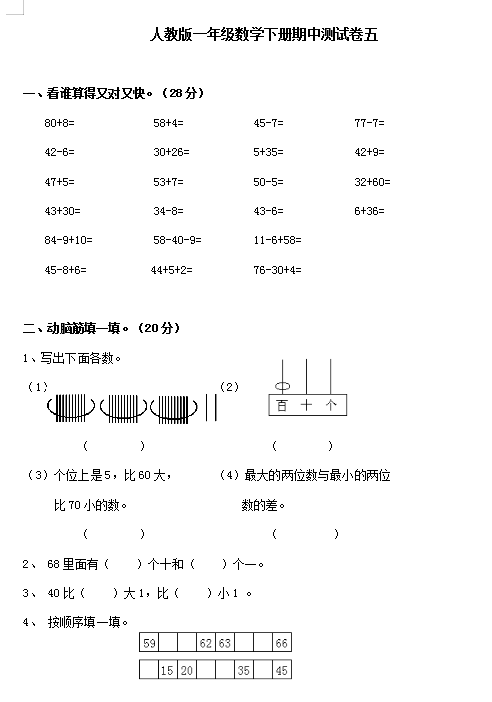80+8=     58+4=    45-7=   77-7=

42-6=     30+26=    5+35=   42+9=

47+5=     53+7=    50-5=   32+60=

43+30=     34-8=    43-6=   6+36=

84-9+10=     58-40-9=    11-6+58=

45-8+6= 44+5+2=    76-30+4=